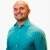# SAT Prep How to determine the the missing value by setting up two proportionsThis lesson will look at an example problem involving proportions. This lesson will give an explanation of how to determine the the missing value by setting up two proportions with a step by step look to... This lesson will look at an example problem involving proportions. This lesson will give an explanation of how to determine the the missing value by setting up two proportions with a step by step look to better explain the concept.
More... Collapse
6 Views